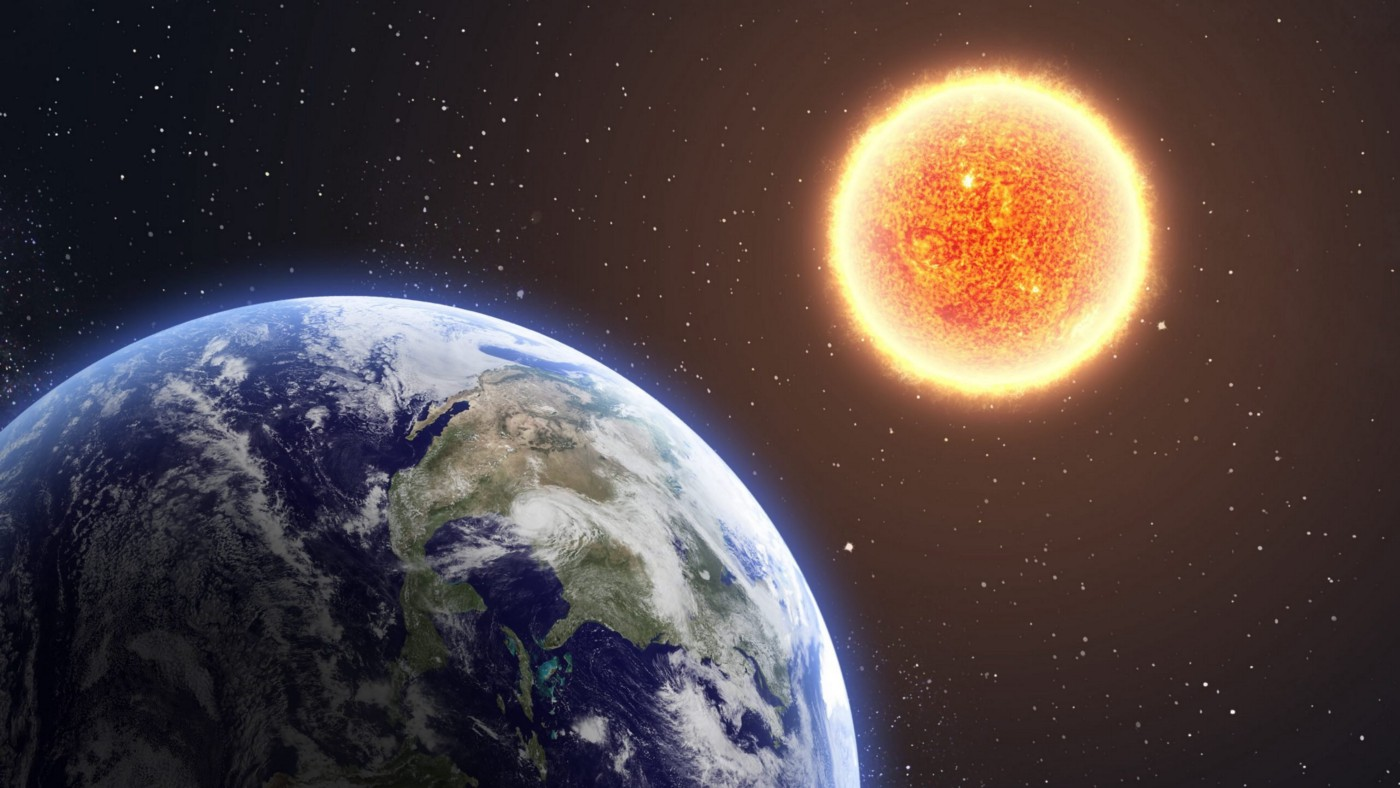# Centripetal Force Study Guide

Introduction

Have you ever considered how the earth revolves around the sun? The only reason the earth isn’t flying through space in a straight line (which would turn us all into human popsicles) is because of the gravity and the centripetal force of our sun. Let’s study this centripetal force in detail.Source

To change the state of an object from rest or motion, you need force, and force behaves differently on different things depending on what type of motion they display. In the situation of curvilinear motion, a particular force called a centripetal force – literally “center seeking” – enters the scene.

## CENTRIPETAL FORCE

Centripetal force’s definition says that it is the force operating on a body to bring it closer to the center of the circular path it is rotating on.

We can also say that an object moving in uniform circular motion experiences an acceleration toward the center of the circular path. Hence they must also experience a force toward the center of the circle. The centripetal force is that force. Being a force, the SI unit of this is Newtons.

The direction of a centripetal force of an item moving on a circular path always works towards the center of the circular path, according to Newton’s second law of motion.

## CALCULATING CENTRIPETAL FORCE

F=ma, or Newton’s second law, represents the relationship between force and acceleration.

Also, we know that centripetal acceleration ( angular acceleration here) is ar = V2/R

By using this value of acceleration (ar ) in the expression of newton’s second law, we can get the centripetal force’s formula, which is

Fc = mV2/R,

Where Fc is the centripetal force,

m is the mass,

V is the speed or velocity,

# SUMMARY

• Centripetal force is a “center-seeking” force that always directs to the rotational center.
• The direction of the centripetal force is towards the center of the circular path.

## FAQs

Q. Can centripetal force point up?

No, centripetal force will never point up; its direction is always towards the center of the circular path it is rotating in.

Q. What is centripetal force affected by?

Mass, speed, and radius are the three factors that affect centripetal force.

Q. Is centripetal force always positive?

The force will only be directed in one direction, towards the center. There is no negative since there is no direct opposite for this force by definition.

Q. Can a centripetal force work on an object?

The direction of motion is always perpendicular to the centripetal force. Work can only be done by the portion of the force that is in the direction of motion. Because the centripetal force lacks such a component, it will never be able to perform work.

We hope you enjoyed studying this lesson and learned something cool about the Centripetal Force! Join our Discord community to get any questions you may have answered and to engage with other students just like you! We promise, it makes studying much more fun!😎

## REFERENCE

1. Centripetal Force: https://flexbooks.ck12.org/cbook/ck-12-physics-flexbook-2.0/section/5.2/primary/lesson/centripetal-force-phys/. Accessed 11th April 2022.
2. What is Centripetal Force? https://www.khanacademy.org/science/physics/centripetal-force-and-gravitation/centripetal-forces/a/what-is-centripetal-force. Accessed 11th April 2022
]]>